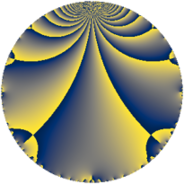# Properties

 Label 4900.2.a.eLevel $4900$ Weight $2$ Character orbit 4900.a Self dual yes Analytic conductor $39.127$ Analytic rank $1$ Dimension $1$ CM no Inner twists $1$

# Related objects

## Newspace parameters

 Level: $$N$$ $$=$$ $$4900 = 2^{2} \cdot 5^{2} \cdot 7^{2}$$ Weight: $$k$$ $$=$$ $$2$$ Character orbit: $$[\chi]$$ $$=$$ 4900.a (trivial)

## Newform invariants

 Self dual: yes Analytic conductor: $$39.1266969904$$ Analytic rank: $$1$$ Dimension: $$1$$ Coefficient field: $$\mathbb{Q}$$ Coefficient ring: $$\mathbb{Z}$$ Coefficient ring index: $$1$$ Twist minimal: no (minimal twist has level 20) Fricke sign: $$1$$ Sato-Tate group: $\mathrm{SU}(2)$

## $q$-expansion

 $$f(q)$$ $$=$$ $$q - 2 q^{3} + q^{9} + O(q^{10})$$ $$q - 2 q^{3} + q^{9} + 2 q^{13} - 6 q^{17} + 4 q^{19} - 6 q^{23} + 4 q^{27} + 6 q^{29} + 4 q^{31} - 2 q^{37} - 4 q^{39} - 6 q^{41} + 10 q^{43} - 6 q^{47} + 12 q^{51} + 6 q^{53} - 8 q^{57} - 12 q^{59} - 2 q^{61} - 2 q^{67} + 12 q^{69} - 12 q^{71} + 2 q^{73} + 8 q^{79} - 11 q^{81} + 6 q^{83} - 12 q^{87} + 6 q^{89} - 8 q^{93} + 2 q^{97} + O(q^{100})$$

## Embeddings

For each embedding $$\iota_m$$ of the coefficient field, the values $$\iota_m(a_n)$$ are shown below.

For more information on an embedded modular form you can click on its label.

Label $$\iota_m(\nu)$$ $$a_{2}$$ $$a_{3}$$ $$a_{4}$$ $$a_{5}$$ $$a_{6}$$ $$a_{7}$$ $$a_{8}$$ $$a_{9}$$ $$a_{10}$$
1.1
 0
0 −2.00000 0 0 0 0 0 1.00000 0
 $$n$$: e.g. 2-40 or 990-1000 Significant digits: Format: Complex embeddings Normalized embeddings Satake parameters Satake angles

## Atkin-Lehner signs

$$p$$ Sign
$$2$$ $$-1$$
$$5$$ $$1$$
$$7$$ $$-1$$

## Inner twists

This newform does not admit any (nontrivial) inner twists.

## Twists

By twisting character orbit
Char Parity Ord Mult Type Twist Min Dim
1.a even 1 1 trivial 4900.2.a.e 1
5.b even 2 1 980.2.a.h 1
5.c odd 4 2 4900.2.e.f 2
7.b odd 2 1 100.2.a.a 1
15.d odd 2 1 8820.2.a.g 1
20.d odd 2 1 3920.2.a.h 1
21.c even 2 1 900.2.a.b 1
28.d even 2 1 400.2.a.c 1
35.c odd 2 1 20.2.a.a 1
35.f even 4 2 100.2.c.a 2
35.i odd 6 2 980.2.i.i 2
35.j even 6 2 980.2.i.c 2
56.e even 2 1 1600.2.a.w 1
56.h odd 2 1 1600.2.a.c 1
84.h odd 2 1 3600.2.a.be 1
105.g even 2 1 180.2.a.a 1
105.k odd 4 2 900.2.d.c 2
140.c even 2 1 80.2.a.b 1
140.j odd 4 2 400.2.c.b 2
280.c odd 2 1 320.2.a.f 1
280.n even 2 1 320.2.a.a 1
280.s even 4 2 1600.2.c.d 2
280.y odd 4 2 1600.2.c.e 2
315.z even 6 2 1620.2.i.b 2
315.bg odd 6 2 1620.2.i.h 2
385.h even 2 1 2420.2.a.a 1
420.o odd 2 1 720.2.a.h 1
420.w even 4 2 3600.2.f.j 2
455.h odd 2 1 3380.2.a.c 1
455.u even 4 2 3380.2.f.b 2
560.be even 4 2 1280.2.d.g 2
560.bf odd 4 2 1280.2.d.c 2
595.b odd 2 1 5780.2.a.f 1
595.u odd 4 2 5780.2.c.a 2
665.g even 2 1 7220.2.a.f 1
840.b odd 2 1 2880.2.a.f 1
840.u even 2 1 2880.2.a.m 1
1540.b odd 2 1 9680.2.a.ba 1

By twisted newform orbit
Twist Min Dim Char Parity Ord Mult Type
20.2.a.a 1 35.c odd 2 1
80.2.a.b 1 140.c even 2 1
100.2.a.a 1 7.b odd 2 1
100.2.c.a 2 35.f even 4 2
180.2.a.a 1 105.g even 2 1
320.2.a.a 1 280.n even 2 1
320.2.a.f 1 280.c odd 2 1
400.2.a.c 1 28.d even 2 1
400.2.c.b 2 140.j odd 4 2
720.2.a.h 1 420.o odd 2 1
900.2.a.b 1 21.c even 2 1
900.2.d.c 2 105.k odd 4 2
980.2.a.h 1 5.b even 2 1
980.2.i.c 2 35.j even 6 2
980.2.i.i 2 35.i odd 6 2
1280.2.d.c 2 560.bf odd 4 2
1280.2.d.g 2 560.be even 4 2
1600.2.a.c 1 56.h odd 2 1
1600.2.a.w 1 56.e even 2 1
1600.2.c.d 2 280.s even 4 2
1600.2.c.e 2 280.y odd 4 2
1620.2.i.b 2 315.z even 6 2
1620.2.i.h 2 315.bg odd 6 2
2420.2.a.a 1 385.h even 2 1
2880.2.a.f 1 840.b odd 2 1
2880.2.a.m 1 840.u even 2 1
3380.2.a.c 1 455.h odd 2 1
3380.2.f.b 2 455.u even 4 2
3600.2.a.be 1 84.h odd 2 1
3600.2.f.j 2 420.w even 4 2
3920.2.a.h 1 20.d odd 2 1
4900.2.a.e 1 1.a even 1 1 trivial
4900.2.e.f 2 5.c odd 4 2
5780.2.a.f 1 595.b odd 2 1
5780.2.c.a 2 595.u odd 4 2
7220.2.a.f 1 665.g even 2 1
8820.2.a.g 1 15.d odd 2 1
9680.2.a.ba 1 1540.b odd 2 1

## Hecke kernels

This newform subspace can be constructed as the intersection of the kernels of the following linear operators acting on $$S_{2}^{\mathrm{new}}(\Gamma_0(4900))$$:

 $$T_{3} + 2$$ $$T_{11}$$ $$T_{13} - 2$$ $$T_{19} - 4$$ $$T_{23} + 6$$

## Hecke characteristic polynomials

$p$ $F_p(T)$
$2$ $$T$$
$3$ $$2 + T$$
$5$ $$T$$
$7$ $$T$$
$11$ $$T$$
$13$ $$-2 + T$$
$17$ $$6 + T$$
$19$ $$-4 + T$$
$23$ $$6 + T$$
$29$ $$-6 + T$$
$31$ $$-4 + T$$
$37$ $$2 + T$$
$41$ $$6 + T$$
$43$ $$-10 + T$$
$47$ $$6 + T$$
$53$ $$-6 + T$$
$59$ $$12 + T$$
$61$ $$2 + T$$
$67$ $$2 + T$$
$71$ $$12 + T$$
$73$ $$-2 + T$$
$79$ $$-8 + T$$
$83$ $$-6 + T$$
$89$ $$-6 + T$$
$97$ $$-2 + T$$